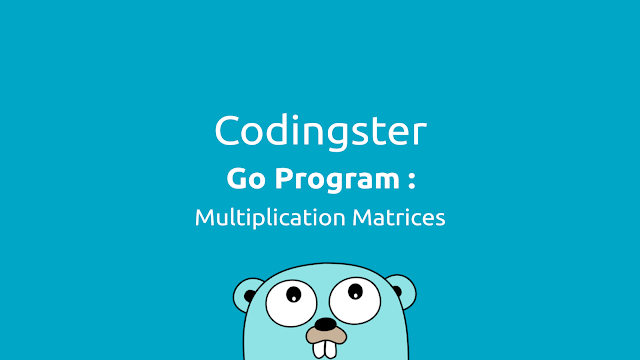-->

## Social Items

Go Program To Multiplication Two Matrices (Golang) - In this post, we will learn how to create a program calculate the two matrix multiplication in the Go programming language.

Matrix is a collection of numbers arranged in rows (vertical) and columns (horizontal) can also be called two-dimensional arrays. matrix multiplication has the condition that the number of columns of the first matrix is equal to the number of rows of the second matrix.

## Go Program To Multiplication Two Matrices (Golang)

### Source Code:

``````package main

import "fmt"

func main() {

var matrixA int
var matrixB int
var result int
var i, j, k, m, n, p, q, total int

total = 0

fmt.Print("Enter the number of rows the first matrix: ")
fmt.Scanln(&m)
fmt.Print("Enter the number of columns the first matrix: ")
fmt.Scanln(&n)

fmt.Print("Enter the number of rows the second matrix: ")
fmt.Scanln(&p)
fmt.Print("Enter the number of columns the second matrix : ")
fmt.Scanln(&q)

if n != p {
fmt.Println("Error: The matrix cannot be multiplied")
} else {

fmt.Println("Enter the first matrix elements: ")
for i = 0; i < m; i++ {
for j = 0; j < n; j++ {
fmt.Scan(&matrixA[i][j])
}
}

fmt.Println("Enter the second matrix elements: ")
for i = 0; i < p; i++ {
for j = 0; j < q; j++ {
fmt.Scan(&matrixB[i][j])
}
}

for i = 0; i < m; i++ {
for j = 0; j < q; j++ {
for k = 0; k < p; k++ {
total = total + matrixA[i][k]*matrixB[k][j]
}
result[i][j] = total
total = 0
}
}

fmt.Println("Results of matrix multiplication: ")
for i = 0; i < m; i++ {
for j = 0; j < n; j++ {
fmt.Print(result[i][j], "\t")
}
fmt.Print("n")
}
}
}
``````

Save the source code with the name of multiplymatrices.go, but adjust wrote with a file name that chills and don't forget the extension should .go

### Compile & Run :

Here's how to compile source code manually:
``````
\$ go build multiplymatrices.go
\$ ./multiplymatrices
``````
You can run without having to compile it:
``````
\$ go run multiplymatrices.go
``````

### The Output of Program :Picture of result the program

### Conclusion:

From the results of the matrix multiplication program, it can be successfully run without any errors and display the results of the first matrix multiplication and the second matrix with a 3 x 3 order.## Go Program To Multiplication Two Matrices (Golang)

Go Program To Multiplication Two Matrices (Golang) - In this post, we will learn how to create a program calculate the two matrix multiplication in the Go programming language.

Matrix is a collection of numbers arranged in rows (vertical) and columns (horizontal) can also be called two-dimensional arrays. matrix multiplication has the condition that the number of columns of the first matrix is equal to the number of rows of the second matrix.

## Go Program To Multiplication Two Matrices (Golang)

### Source Code:

``````package main

import "fmt"

func main() {

var matrixA int
var matrixB int
var result int
var i, j, k, m, n, p, q, total int

total = 0

fmt.Print("Enter the number of rows the first matrix: ")
fmt.Scanln(&m)
fmt.Print("Enter the number of columns the first matrix: ")
fmt.Scanln(&n)

fmt.Print("Enter the number of rows the second matrix: ")
fmt.Scanln(&p)
fmt.Print("Enter the number of columns the second matrix : ")
fmt.Scanln(&q)

if n != p {
fmt.Println("Error: The matrix cannot be multiplied")
} else {

fmt.Println("Enter the first matrix elements: ")
for i = 0; i < m; i++ {
for j = 0; j < n; j++ {
fmt.Scan(&matrixA[i][j])
}
}

fmt.Println("Enter the second matrix elements: ")
for i = 0; i < p; i++ {
for j = 0; j < q; j++ {
fmt.Scan(&matrixB[i][j])
}
}

for i = 0; i < m; i++ {
for j = 0; j < q; j++ {
for k = 0; k < p; k++ {
total = total + matrixA[i][k]*matrixB[k][j]
}
result[i][j] = total
total = 0
}
}

fmt.Println("Results of matrix multiplication: ")
for i = 0; i < m; i++ {
for j = 0; j < n; j++ {
fmt.Print(result[i][j], "\t")
}
fmt.Print("n")
}
}
}
``````

Save the source code with the name of multiplymatrices.go, but adjust wrote with a file name that chills and don't forget the extension should .go

### Compile & Run :

Here's how to compile source code manually:
``````
\$ go build multiplymatrices.go
\$ ./multiplymatrices
``````
You can run without having to compile it:
``````
\$ go run multiplymatrices.go
``````

### The Output of Program :Picture of result the program

### Conclusion:

From the results of the matrix multiplication program, it can be successfully run without any errors and display the results of the first matrix multiplication and the second matrix with a 3 x 3 order.